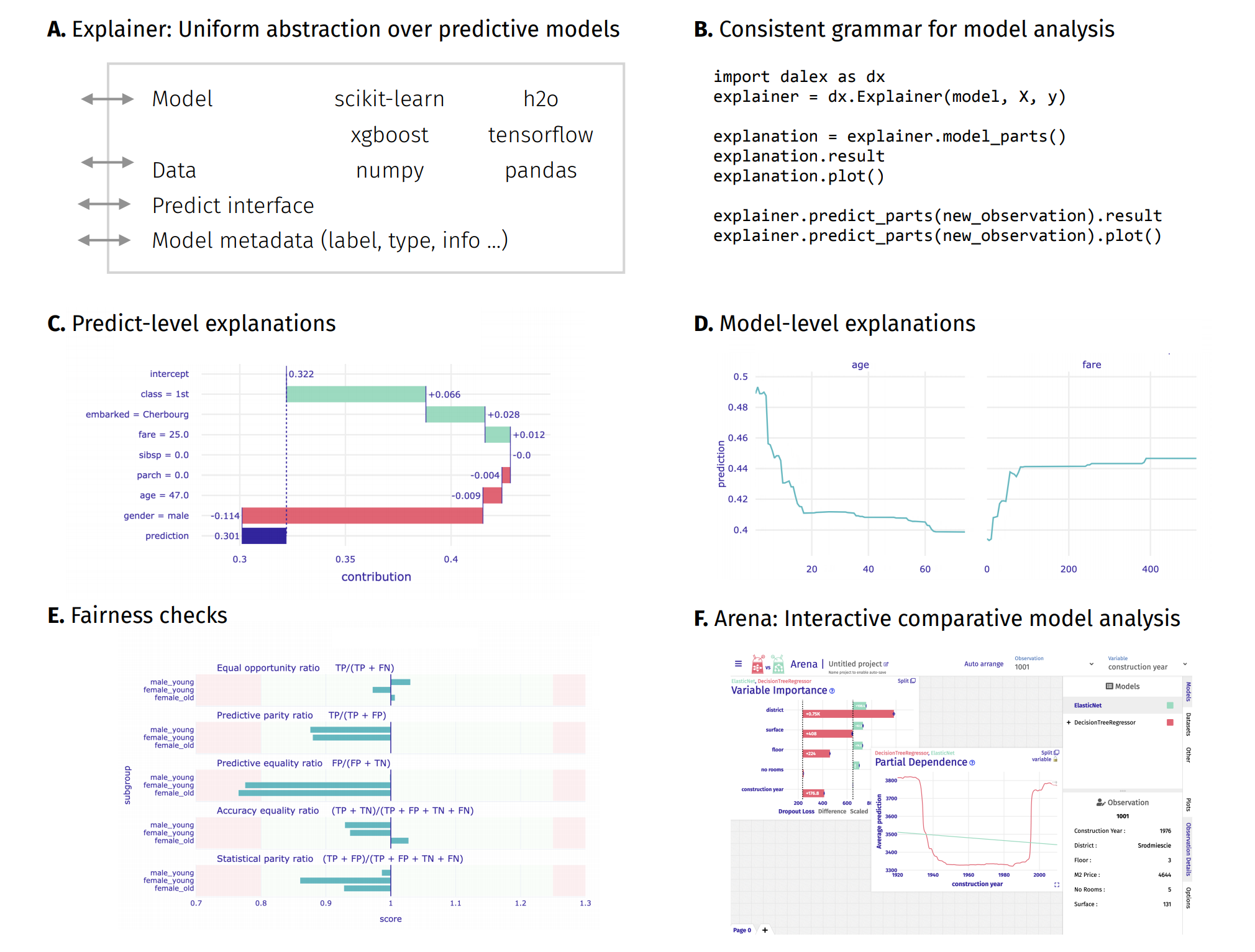# Titanic example for xgboost¶

### introduction to the topic: Explanatory Model Analysis: Explore, Explain, and Examine Predictive Models¶### Imports¶

In :
import dalex as dx

import pandas as pd
import numpy as np

from sklearn.neural_network import MLPClassifier
from sklearn.preprocessing import StandardScaler, OneHotEncoder
from sklearn.impute import SimpleImputer
from sklearn.pipeline import Pipeline
from sklearn.compose import ColumnTransformer

import xgboost as xgb

import warnings
warnings.filterwarnings('ignore')

In :
dx.__version__

Out:
'1.4.0'

# Basic example with xgb.train¶

We will consider the most basic example first. Due to categorical variables in the dataset, that are not handled by default by xgb, we will drop them in the first example.

In :
data = dx.datasets.load_titanic()

X = data.drop(columns='survived').loc[:, ['age', 'fare', 'sibsp', 'parch']]
y = data.survived

In :
params = {
"max_depth": 5,
"objective": "binary:logistic",
"eval_metric": "auc"
}

train = xgb.DMatrix(X, label=y)
classifier = xgb.train(params, train, verbose_eval=1)


Note that despite special data format needed by the xgb, you pass pandas.DataFrame to the Explainer. You have to use Data Frame in all interactions with the Explainer.

In :
exp = dx.Explainer(classifier, X, y)

Preparation of a new explainer is initiated

-> data              : 2207 rows 4 cols
-> target variable   : Parameter 'y' was a pandas.Series. Converted to a numpy.ndarray.
-> target variable   : 2207 values
-> model_class       : xgboost.core.Booster (default)
-> label             : Not specified, model's class short name will be used. (default)
-> predict function  : <function yhat_xgboost at 0x00000211FD366790> will be used (default)
-> predict function  : Accepts only pandas.DataFrame, numpy.ndarray causes problems.
-> predicted values  : min = 0.0766, mean = 0.328, max = 0.881
-> model type        : 'model_type' not provided and cannot be extracted.
-> model type        : Some functionalities won't be available.
-> residual function : difference between y and yhat (default)
-> residuals         : min = -0.83, mean = -0.006, max = 0.847
-> model_info        : package xgboost

A new explainer has been created!


Again, note that X is just a Data Frame.

In :
exp.predict(X)

Out:
array([0.2206684 , 0.6000112 , 0.4254955 , ..., 0.26790908, 0.23142426,
0.25531068], dtype=float32)
In :
exp.model_parts().plot()